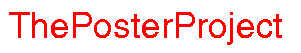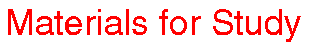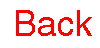Visualizing Women in Science, Mathematics and Engineering
• Home
• Posters
• Materials for Study
• Biographies
• The Design Team
•# Time and the Number Theorist

Number theory deals with the magical properties of integers: divisibility, factorizations, prime numbers. We'll look at some of these issues.

There is an amazing detail of pattern hidden in numbers. Check out the following sequences of numbers, and see if you can work out what the next entry should be:

• 1, 9, 36, 100, .. ? ..
• 26, 37, 61, 100, .. ?..
• 37, 41, 43, 47, 53, .. ? ..
• 24, 120, 720, 5040, .. ? ..
• 1, 11, 21, 1211, 111221, 312211, .. ? ..

One of the most important aspect of numbers (and here by numbers I mean non-negative integers) is that they can be written as the product of prime numbers. A prime number is a number which has exactly two factors: 1 and itself. (Notice that by this definition, 1 is not a prime number. Why?)

Here is a way to find all the prime numbers less than 100.

## The Sieve of Erastothenes

• Draw a 10x10 table and, starting with 1 in the top left corner, moving right across the row, enter the first 100 numbers.
• Now get a big red pen (okay it doesn't have to be red, any nice bright color will work).
• Cross out 1.
• What are the factors of 2? Well, the only factors of 2 are 2 and 1, so 2 is prime. We can't cross that out. But any number that is divisible by 2 has to go. In other words, we have to kill all the even numbers. Cross out the whole column under 2 (but not 2!), and all of the columns headed by 4, 6, 8 and 10. Wow. That took care of quite a bit.
• What's the next number we haven't crossed out? It is 3. Is 3 prime? Yes. (Why?) Leave 3, but cross out all its multiples.
• Now go on to the next number we haven't looked at and which isn't crossed out (it should be 5) and repeat the process.
• Keep going until you get to 97, the biggest prime less than 100.

Of course, there is no reason why this method should be limited to numbers less than 100. It can get difficult to do the arithmetic by hand, but luckily we have powerful computers. Here are two computer projects:

• Write a computer program which implements the Sieve of Erastothenes for all numbers less than 1000. How about for numbers less than a million?
• Write a computer program which decides if a given number is prime. How big a number are you going to allow to be inputed? How efficient can you make your program?

If you'd like to know more about primes, here is another cool site: the prime page.

Let's look a little bit closer at divisibility.

## Divisibility rules

Before the advent of calculators and computers (you know, when dinosaurs roamed the earth), people needed a quick way to know if a given number was divisible by, say, 3. Take 54879731112156. Is it divisible by 3? Is there a quick way of knowing, without having to do the division?

Can you work out the trick to see if a number is divisible by 2?

Now let's think about 3. Is 23 divisible by 3? There goes that trick. We'll have to think of something a little cleverer. How about a trick for 4? Let me state the problem clearly:

Think up short-cut techniques for deciding if a given number is divisible by 2,3,4,5,6,7,8,9,or 10.
When you're done, you can check my answers.

All this talk about division reminds me of my favorite scene in a movie called Little Man Tate . The movie was Jodie Foster's directorial debut and is the story of a young math genius, and the difficulties he has being "different" from everyone else. Anyway, he is sitting in math class pretty much doing his own thing, while the teacher hovers at the board. The numbers 1 through 10 are written on the board, and the teacher implores the class to tell her which of these numbers is divisible by 2. No one will answer. Eventually, desperate, she calls on little man Tate. Without looking up, he answers, "All of them."

Can you see why he was right?

He was right because there is a difference between being exactly divisible by 2 and just being divisible by 2. 3, for example, is divisible by 2, with a remainder of 1.

Sometimes it is the remainder which we are interested in.

## Modular Arithmetic

Let's look at a clock.
[picture of clock]

A clock is numbered from 1 to 12. Suppose it is 10 o'clock and I want to know what the time will be three hours from now. Well, this is not rocket science. If it is now 10 then in three hours time it will be 1 o'clock. Let's write this down a little differently.
10+3=1
That is a little different!

Let's see if we can understand exactly where this 1 is coming from. The first thing to understand is that we are working modulo 12, because there are exactly 12 hours. (Modulo comes from Latin and means "with respect to". Another way of thinking about it would be to think of the word "base" as in "base 10" -- our normal decimal system. Anyway, working modulo 12 means "working with respect to 12".) Now normally,

10+3=13
so what we are really asking is: what is
13 modulo 12
which we abbreviate (mathematicians are notoriously lazy!) as
13 mod 12
Now we already know what we want this to be:
13 mod 12 =1

Let's try a few more examples. Suppose it is now 5 o'clock. In four hours it will be 9 o'clock. That is:

5+4=9
or
5+4= 9 mod 12
or
9=9 mod 12
And one more example. If it is now 2 o'clock, then in fifteen hours it will be 5 o'clock. Writing this down gives:
2+15=5
or
5= 17 mod 12

Can you work out the rule?

Here are some things to think about:
• How many possibilities are there for a mod 12?
• How many possibilities are there for a mod n?
• Show that any integer is equal to the sum of its decimal digits mod 9 (for example: 41 mod 9= 5 and (4+1) mod 9=5 mod 9 = 5, 28 mod 9 =1 and (2+8) mod 9 = 10 mod 9 =1.)
• Think of other examples from daily life where we use modular arithmetic.

If you like number theory, you should check out the Golden Mean poster. You can also read more about number theory in the Mathematical Atlas.